/

### The non-central t distribution

 Distribution name (Standard) non-central t distribution (NCT) Common notation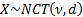Parameters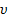= degrees of freedom (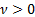, usually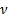is an integer although in some situations a non-integralcan arise)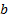= non-centrality parameter Domain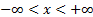Probability density function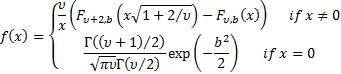where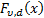is the NCT cumulative distribution function Cumulative distribution function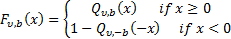where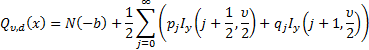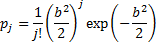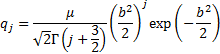Mean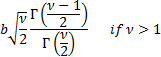Variance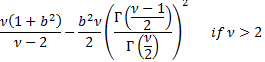Other comments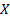has a standard non-centraldistribution withdegrees of freedom and non-centrality parameter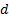if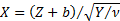,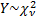,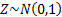and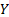and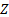are independent. It has the following non-central moments: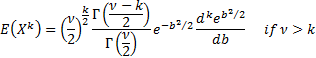Nematrian web functions

Functions relating to the above distribution may be accessed via the Nematrian web function library by using a DistributionName of “non-central t”. Functions relating to a generalised version of this distribution including additional location (i.e. shift) and scale parameters may be accessed by using a DistributionName of “non-central t4”, see also including additional shift and scale parameters. For details of other supported probability distributions see here.